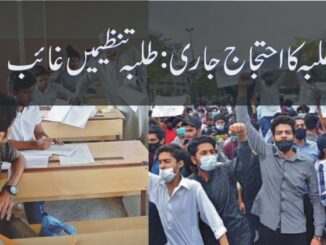Library Sindh Study FG Study Punjab Study# SAMPLE QUESTIONS CHEMISTRY Quaid-e-Azam Medical College Pakistan

####Abdullah gul##### SAMPLE QUESTIONS CHEMISTRY Quaid-e-Azam Medical College Pakistan
« on: August 23, 2009, 03:01:10 PM »
SAMPLE QUESTIONS CHEMISTRY Quaid-e-Azam Medical College Pakistan

9. Which of the following compound of CI contain ionic bonds only?

A. NaCI
B. CHCl3
C. NCI3
D. CCI4

10. The empirical formula of a liquid compound is C6 H12 O6. To find molecular formula, it is necessary to know the

A. Boiling point of the compound
B. Density of the compound
C. Relative molecular mass of the compound
D. Percentage composition of the compound

11. Isotopes have the same

A. Electron structure
B. Mass number
C. Number of neutrons
D. Physical properties

12. The rate of evaporation of water can be decreased by

A. Raising the temperature of water
B. Exposing the surface area to the air
C. Increasing the pressure of the surrounding air
D. Keeping the surrounding air dry

13. The chemistry of Carbon is called

A. Organic Chemistry
B. Inorganic Chemistry
C. Physical Chemistry
D. Pharmaceutical Chemistry

14. What volume of carbon dioxide would be produced if 5 litres of propane, C3 H8  were burnt in air according to the following equation

C3H8+5O2 = 4H2O + 3CO2

A. One litre
B. Three litres
C. Fifteen litres
D. Thirty litres